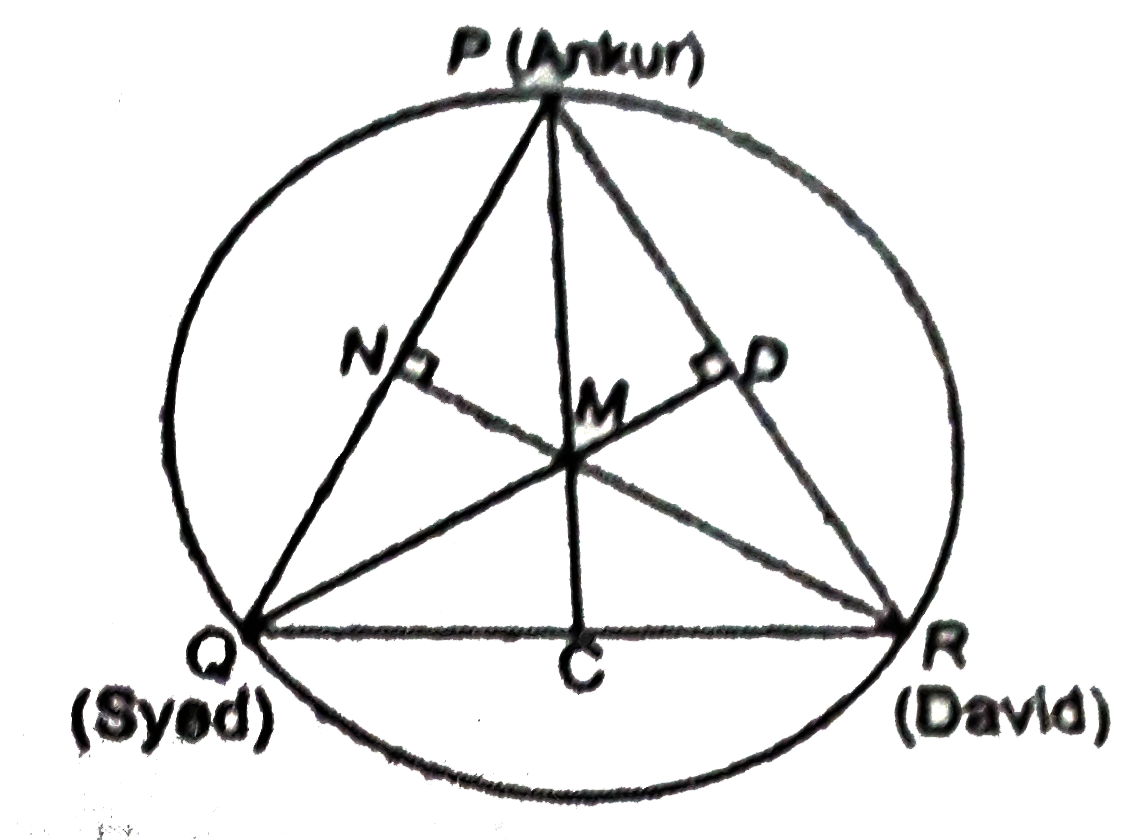# A circular park of radius 20m is situated in a colony. Three boys Ankur, Syed and David are sitting at equal distance on its boundary each having a to

57 views

closed
A circular park of radius 20m is situated in a colony. Three boys Ankur, Syed and David are sitting at equal distance on its boundary each having a toy telephone in his hands to talk each other. Find the length of the string of each phone.

by (88.7k points)
selected by

Let Ankur, Syed and David standing on the points P,Q and R.
Let PQ=QR=PR=x
Therefore, DeltaPQR is an equilateral triangle. Drawn altitudes PC, OD and RN form vertices to the sides of a triangle and intersect these altitude at the centre of a circle M.
As PQR is an equilateral, therefore these altitude bisects their sides.
In DeltaPQC
PQ^2=PC^2+QC^2 (by pythagorus theorem)
x^2=PC^2+((x)/(2))^2
rArr PC^2=x^(2)-(x^2)/(4)=(3x^2)/(4)" "(because QC=(1)/(2)QR=(x)/2)
rArrPC=sqrt(3x)/(2)
Now, MC=PC-PM=sqrt(3x)/(2)-20" "(becausePM="radius"=20 m)
In DeltaQCM,
QM^2=QC^2+MC^2
(20)^2=(x/2)^2+(sqrt(3x)/(2)-20)^2" "(because QM ="radius"=20 m)
rArr400=(x^2)/(4)+-20sqrt(3x)+400
rArr0=x^2-20sqrt(3x)
rArrx=20sqrt(3)m
HEnce, PQ=QR =PS= 20(sqrt3)m.